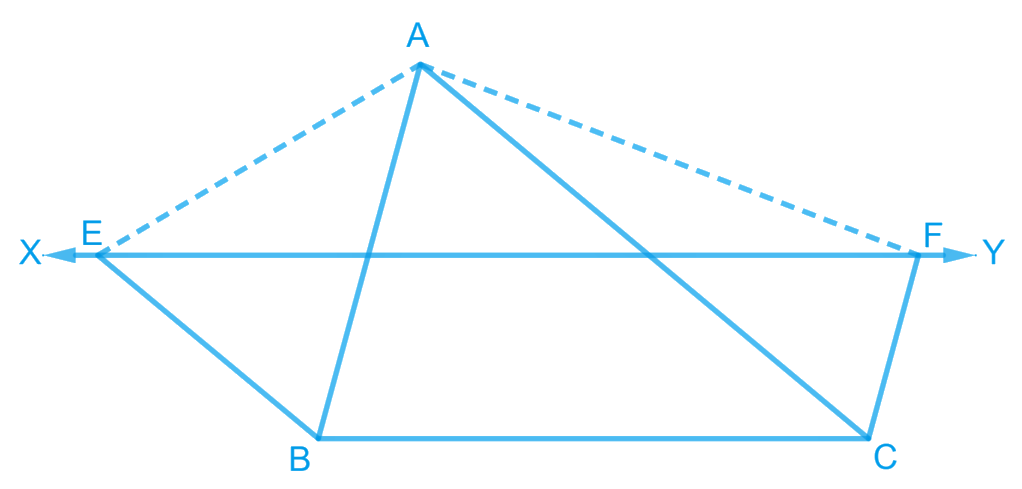# Ex.9.3 Q8 Areas of Parallelograms and Triangles Solution - NCERT Maths Class 9

Go back to  'Ex.9.3'

## Question

$$XY$$ is a line parallel to side $$BC$$ of a triangle $$ABC$$. If $$BE\; || \;AC$$ and $$CF\; || \;AB$$ meet $$XY$$ at $$E$$ and $$F$$ respectively, show that $$ar (ABE) = ar (ACF)$$

Video Solution
Areas Of Parallelograms And Triangles
Ex 9.3 | Question 8

## Text Solution

What is known?

$$XY$$ is a line parallel to side $$BC$$ of a triangle $$ABC$$. $$BE || AC$$ and $$CF || AB$$ meet $$XY$$ at $$E$$ and $$F$$ respectively.

What is unknown?

How we can show that $$ar (ABE) = ar (ACF).$$

Reasoning:

if a triangle and parallelogram are on same base and between same parallel lines then area of triangle will be half of area of parallelogram. Also, if two quadrilaterals are on same base and between same pair of parallel lines then both will have equal area. By using these theorems, we can show the required result.

Steps:

Let’s draw points $$M$$ and $$N$$, intersected by line $$XY$$ on sides $$AB$$ and $$AC$$ respectively.It is given that,

$$XY || BC$$ and

$$BE || AC$$

Hence, $$EN || BC$$ and $$BE || CN$$

Therefore, $$BCNE$$ is a parallelogram

It is given that

$$XY || BC$$ and $$FC || AB$$

Hence, $$MF || BC$$ and $$FC || MB$$

Therefore, $$BCFM$$ is a parallelogram.

Parallelograms $$EBCY$$ and $$BCFX$$ are on the same base $$BC$$ and between the same parallels $$BC$$ and $$EF$$.

According to Theorem 9.1 : Parallelograms on the same base and between the same parallels are equal in area.

Therefore

\begin{align}\text {ar}({EBCY})=\text {ar}({BCFX})...\!(1)\end{align}

Consider parallelogram  and $$\Delta AEB$$

These lie on the same base  and are between the same parallels  and .

Therefore\begin{align}\text {ar}(\Delta {ABE})=\frac{1}{2} \text {ar}({EBCY})...\!(2)\end{align}

Also, parallelogram $$\Delta CFXB$$ and $$\Delta ACF$$ are on the same base  and between the same parallels  and .

Therefore

\begin{align}\text {ar}(\Delta {ACF})=\frac{1}{2} \text {ar}({BCFX})...\!(3)\end{align}

From Equations (), (), and (), we obtain

Area ($$\Delta ABE$$)  Area  ($$\Delta ACF$$)

Video Solution
Areas Of Parallelograms And Triangles
Ex 9.3 | Question 8